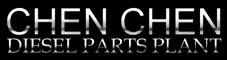E-mail : Sale@dieselpartsworld.com | WhatsApp : +86 13850261121English Español Русский Français Português Polski Italiano Deutsch Türkçe العربية Home Products News F.A.Q Links E-mail : Sale@dieselpartsworld.com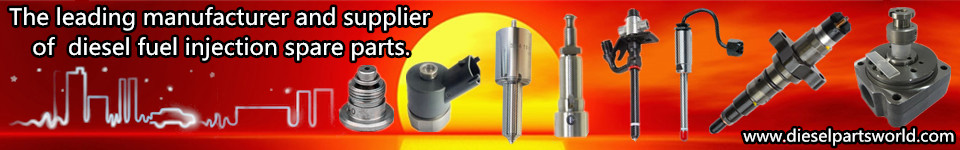Home>> News

News Center :

 Horsepower is a kind of "power"  (Dieselpartsworld) 2016-01-17    Horsepower is a kind of "power" Learn how to turn the torque through the gear box to the actual thrust, then you can study what is called "horsepower.". Horsepower, in fact, is not a "rub", but a kind of power (Power) units, defined as the unit of time to do the "work" of the size of the.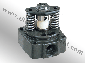However, we have to continue to use the "horsepower" the name, after all, has been used for too long, about "power" I am afraid not a few consumers understand? Power is calculated by the torque, and calculation formula is quite simple: power (W) = 2 pi x torque (n-m) x speed (RPM) / 60, the simplified calculation become: power (kw) = (n-m) of torque x speed (RPM) /9549 and derived in detail please refer to block the. However, how to convert the power of kW to the common "horsepower", which has a story to tell. Inch or metric? 1hp=746W; 1PS=735W The horsepower definition is not the same! When it comes to engine horsepower, I believe many people will intuitively expect what DIN, SAE, EEC, JIS, etc. different testing standards, in the end difference of these standards where. After the empty research; an exaggeration is due to the different inch and metric, the "horsepower" definition basically is not the same. Imperial horsepower (HP) definition: a horse in a minute 200 pounds (LB) of heavy objects pull 165 feet (FT),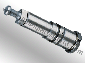the multiplication is equal to 33000ft-lb/min; and metric horsepower (PS) is defined as a horse in a minute 75 kg of objects pull 60 meters, the multiplication is equal to 4500kg-m/min. Through unit conversion, 1lb=0.454kg; 1ft=30.48cm) unexpectedly found 1hp=4566kg-m/min, metric and 1PS=4500kg-m is slightly different, and if the conversion to power W (1W=1Nm/sec= 9.8kgm/sec)! 1hp=746W; 1PS=735W two different results. The same is the "horsepower" definition of imperial horse power and metric horsepower didn't like! Is the British horse more powerful? The world exactly why inch and metric respectively, like why there is a car is driving right, some of it is left to driving, man is always difficult to reconcile the differences. If we are more familiar with several test standard, Germany din and the European Community of new standard of EEC and Japan's JIS is PS with metric units are horsepower, and SAE use is as a unit of the imperial HP, but in order to avoid the complex, this print rate horsepower unit indication for HP. Recently, a growing number of raw plant data has been changed to provide an absolutely non controversial KW as the engine output power value. But then again, the difference between 1HP and 1PS is only 1.5%, one hundred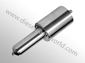horsepower difference of 1.5 horses, the difference is not large. The horsepower of the common saloon car mostly only below the 200 horsepower, both due to the definition of the difference is only a 3 horsepower around, so if you really want to "Mama care", put SAE standard data more than 1.5%. But SAE, JIS, DIN, EEC standard between some slight differences, the old problem has argued many times the, between units can not really equate. However, under the difference is not how much, as the same! So he is PS or HP, can almost be regarded as equal. Finally we can make a conclusion! Will the obtained horsepower and power conversion substitution power and torque conversion formula, and the kg-m torque unit conversion is a familiar, can have the following results: Imperial horsepower hp= torque (kg-m) * (RPM) / 727 rpm Metric horsepower PS = torque (kg-m) x engine speed (RPM) / 716 Do you know what is the use of these formulas? From the horsepower hp= torque x speed /727, it appears that if you can increase the engine speed, torque unchanged, it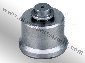can increase the horsepower. For example, if the speed from 6000 to 8000rpm, equivalent to an increase of 33%, but because of camshaft restrictions make 8000rpm torque decreased by 10%, you can still make horsepower increase 19.7%, indicating that the current conversion computer why can dramatically increase horsepower after lift off the oil. So don't be "increased? The advertisement of a horse power. Let us think from another point of view: if the same speed, the increase of 20 horsepower, the number of delegates can increase the thrust of it? The maximum torque point to play in the case of 5000rpm, the formula can be a little change, can be found to increase the torque =20hp x 727 / 5000rpm=2.9kgm. Then the results into the vehicle driving force formula, the same joy beauty a calculation, 2.9 x 3.250 x 4.058/0.41=93 kg. For a ton of body, seems to influence is not very big; furthermore if a difference of 5 horsepower, thrust but only increase 23 kg, visible difference 5 horsepower, there is not much difference, so it can "increase 5 horsepower" pro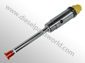ducts, in the end should spend how many money to refit, you alone will handle the?

 Home Products Catalog News Sitemap(xml)